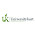### Garmin + Data + R = Combining Hobbies

I'm considering trying to go for the Fastest Known Time at a 60 mile route in South Florida near me. Here is a link to the route I've been looking at. The idea is simple: no official race course, no guides, water stations, aid stations, race fees or any structure - just go run as fast as you can! The current FKT is just under 10 hours...so we'll see.

From the site:

"A near perfect 100 kilometer loop along an Everglades boundary levee in South Florida...Big challenges due to heat, humidity, sun exposure, and effectively no access to drinkable water. Gator sightings are near guaranteed."

I've decided to keep a closer eye on my eye running data to make sure I'm training effectively to run the 60 miles and to identify any potential health concerns before they become serious. Fortunately, by wearing a Garmin watch during all of my training, this data is cleaned and aggregated already - with a significant amount of interesting data points (cadence, distance, calories, time, vertical ratio, etc..).

On Garmin connect it is pretty easy to download all activities as a .csv and then move into R.

### Data Aggregation in R

First, I read in the data and have to perform some simple cleaning
• Remove "," from calories burned
• Remove "," from distance
• Convert date from a timestamp to date
• Aggregate activities to the day level
After cleaning the data, I create some simple calculated fields to be used in the analysis.
• Day of Year (number day)
• Miles Per Hour
• Days since New Year
• Cumulative Distance by Year
• Pace
``````
df = read_csv('Activities.csv', col_names = TRUE,guess_max = 1000)

running = df %>%
filter(`Activity Type` %in% c('Running','Treadmill Running')) %>%
mutate(cleancal = gsub("[^0-9]","",Calories)) %>%
ungroup %>%
mutate(fdate = round_date(Date, unit="day")) %>%
mutate(cleancal = gsub("[^0-9]","",Calories)) %>%
mutate(cleandist = gsub("[,]","",Distance)) %>%
group_by(fdate) %>%
summarize(distance = sum(as.numeric(cleandist)),
cals = sum(as.numeric(cleancal)),
runtime = sum(Time)) %>%
mutate(DayOfYear = strftime(fdate,format="%j")) %>%
mutate(secondtime = as.numeric(runtime)) %>%
mutate(MPH = (distance/secondtime)*60*60) %>% #convert to MPH
mutate(YR = as.factor(year(fdate))) %>%
mutate(NYD = paste(year(fdate),"-01-01",sep="")) %>%
mutate(Days =  ymd(fdate) - ymd(NYD)) %>%
arrange(fdate) %>%
group_by(YR) %>%
mutate(cumsumd = cumsum(distance)) %>%
ungroup %>%
mutate(PACE = (secondtime/60)/distance) %>%
mutate(PACET = paste(floor(PACE), round((PACE-floor(PACE))*60),sep=":")) %>%
mutate(PACET = lubridate::ms(PACET)) %>%
mutate(PACETM = paste(PACET@minute,ifelse(PACET@.Data<10 ata="" paste="" sep=":">%
mutate(PACETM2 = as.POSIXct(strptime(PACETM,format = "%M:%S")))
``````

### Plots!

All of the plots were created using ggplot and the code is at the bottom.

Some of the more interesting takeaways:
• Speed looks to have decreased over the years, and peaked during Ironman Chattanooga training
• I think this is thrown off because I've been much more attentive to using slow long runs for training more recently. The top end speed looks to still be there based on the late 2020 runs!
• 2018 and 2020 are eerily similar in terms of running distance and distance by day
• 2019 was the biggest running year - makes sense given the Ironman training
• Regardless of the distance, pace is relatively flat. This seems to suggest I should be able to run faster on those shorter runs!

###```       ```

#pace (Mph)
p1 = ggplot(data = running, aes(x=fdate, y = MPH, color = distance)) +
geom_point() +
geom_smooth(color = "orange") +
labs(x = "Date", y = "Miles Per Hour",title = "Running Speed Over the Years") +
ylim(6,10) +
#geom_vline(xintercept = ymd('2019-08-03'))
p1   #overall speed trending down, due to intro of slower training runs. More overall high speed runs

#cumulative each year
p2= running %>% group_by(YR) %>% mutate(cumsum = cumsum(distance)) %>%
ggplot(data = ., aes(x=fdate,y=cumsum,group=YR,color=YR)) +
geom_line(lwd=1.3) +
labs(x = "Date", y = "Cumulative Distance (Miles)", title="Cumulative Distance by Year")
p2
#cumulative by each day of year
p3=ggplot(data = running, aes(x=Days,y=cumsumd, group=YR,color=YR)) +
geom_line(lwd=1.4) +
scale_x_continuous() +
labs(x="Days in", y = "Cumulative Distance (Miles)",title="YoY Running Distance")
p3
#pace vs. distnace
p4=ggplot(data=running, aes(x=distance,y=PACETM2,color = fdate)) +
geom_point() +
scale_y_datetime(date_labels = "%M:%S") +
geom_smooth(color="red")+
labs(x="Miles", y = "Pace", title = "Running Distance vs. Pace")
p4
p5=running %>% filter(!fdate == ymd('2017-09-30')) %>%
ggplot(data=., aes(x=distance,y=PACETM2,color = fdate)) +
geom_point() +
scale_y_datetime(date_labels = "%M:%S", limits = c(floor_date(Sys.time(),"day") + 300,
floor_date(Sys.time(),"day") + 700)) +
xlim(2,20) +
geom_smooth(color="red") +
labs(y = "Pace", x = "Miles",title = "Running Distance vs. Pace (Zoom in)")
p5

#save plots
ggsave("mph.png", plot = p1, width = 8, height = 4, dpi = "print")
ggsave("yearcum.png", plot = p2, width = 8, height = 4, dpi = "print")
ggsave("yoy.png", plot = p3, width = 8, height = 4, dpi = "print")
ggsave("distancepace.png", plot = p4, width = 8, height = 4, dpi = "print")
ggsave("zoom_distancepace.png", plot = p5, width = 8, height = 4, dpi = "print")
```
```

1.Thank you for sharing useful information with us. please keep sharing like this. And if you are searching a unique and Top University in India, Colleges discovery platform, which connects students or working professionals with Universities/colleges, at the same time offering information about colleges, courses, entrance exam details, admission notifications, scholarships, and all related topics. Please visit below links:

Mahakaushal University in Jabalpur

YBN University in Ranchi

Manipal University Jaipur

Swami Vivekanand University in Sagar